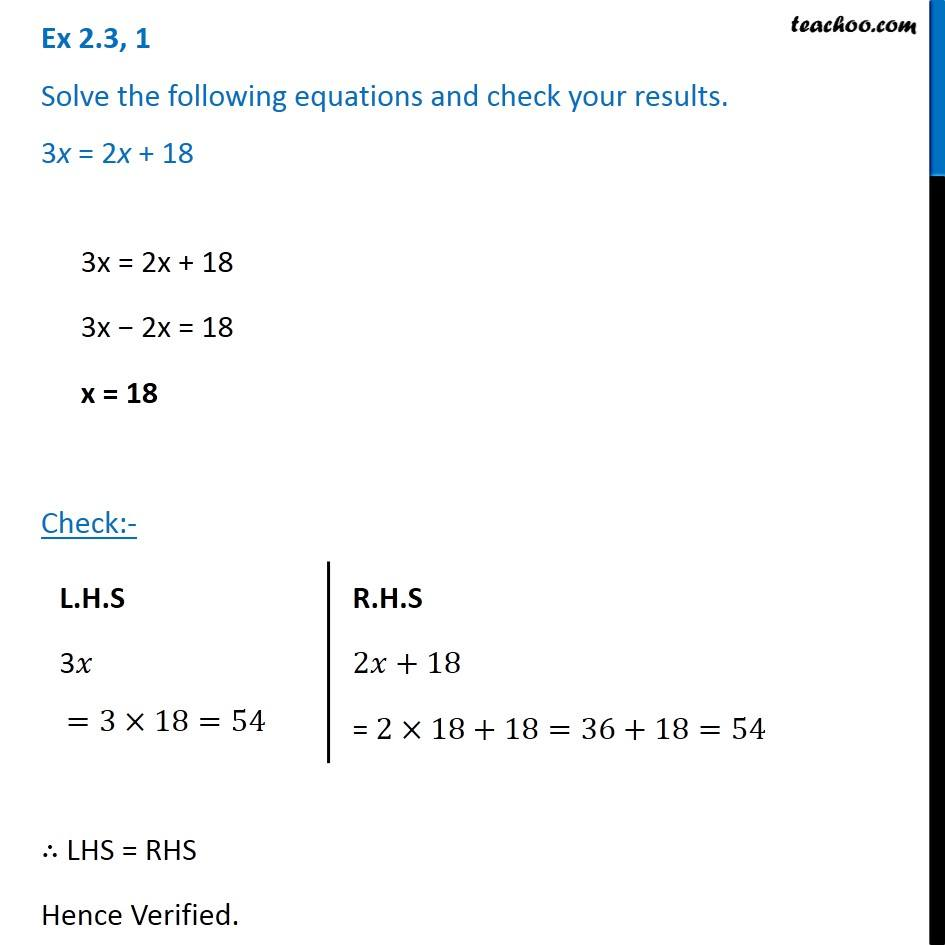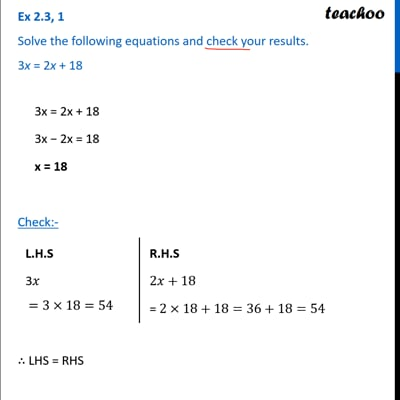Ex 2.3

Chapter 2 Class 8 Linear Equations in One Variable
Serial order wiseThis video is only available for Teachoo black users

Get live Maths 1-on-1 Classs - Class 6 to 12

### Transcript

Ex 2.3, 1 Solve the following equations and check your results. 3x = 2x + 18 3x = 2x + 18 3x − 2x = 18 x = 18 Check:- ∴ LHS = RHS Hence Verified. L.H.S 3𝑥 =3×18=54 R.H.S 2𝑥+18 = 2×18+18=36+18=54